Advertisement Remove all ads

# NCERT solutions for Class 9 Mathematics Textbook chapter 12 - Heron's Formula [Latest edition]

#### Chapters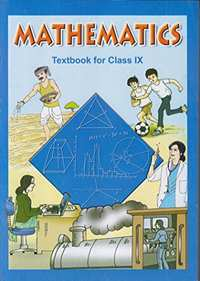Advertisement Remove all ads
Advertisement Remove all ads
Advertisement Remove all ads

## Chapter 12: Heron's Formula

Exercise 12.1Exercise 12.2
Advertisement Remove all ads
Exercise 12.1[Pages 202 - 203]

### NCERT solutions for Class 9 Mathematics Textbook Chapter 12 Heron's FormulaExercise 12.1[Pages 202 - 203]

Exercise 12.1 | Q 1 | Page 202

A traffic signal board, indicating ‘SCHOOL AHEAD’, is an equilateral triangle with side ‘a’. Find the area of the signal board, using Heron’s formula. If its perimeter is 180 cm, what will be the area of the signal board?

Exercise 12.1 | Q 2 | Page 202

The triangular side walls of a flyover have been used for advertisements. The sides of the walls are 122m, 22m, and 120m (see the given figure). The advertisements yield an earning of Rs 5000 per m2per year. A company hired one of its walls for 3 months. How much rent did it pay?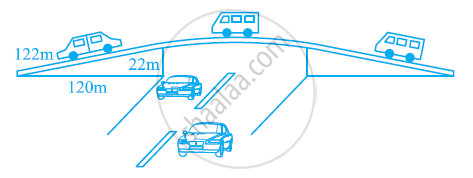Exercise 12.1 | Q 3 | Page 203

There is a slide in the park. One of its side walls has been painted in the same colour with a message “KEEP THE PARK GREEN AND CLEAN” (see the given figure). If the sides of the wall are 15m, 11m, and 6m, find the area painted in colour.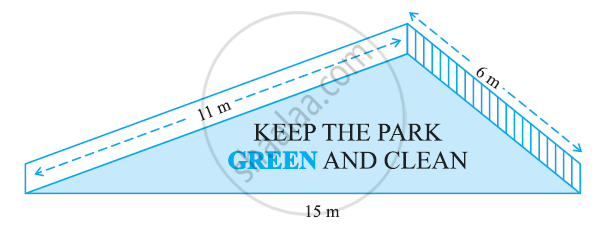Exercise 12.1 | Q 4 | Page 203

Find the area of a triangle two sides of which are 18 cm and 10 cm and the perimeter is 42 cm.

Exercise 12.1 | Q 5 | Page 203

Sides of a triangle are in the ratio of 12: 17: 25 and its perimeter is 540 cm. Find its area.

Exercise 12.1 | Q 6 | Page 203

An isosceles triangle has perimeter 30 cm and each of the equal sides is 12 cm. Find the area of the triangle.

Exercise 12.2[Pages 206 - 207]

### NCERT solutions for Class 9 Mathematics Textbook Chapter 12 Heron's FormulaExercise 12.2[Pages 206 - 207]

Exercise 12.2 | Q 1 | Page 206

A park, in the shape of a quadrilateral ABCD, has ∠C = 90°, AB = 9 m, BC = 12 m, CD = 5 m and AD = 8 m. How much area does it occupy?

Exercise 12.2 | Q 2 | Page 206

Find the area of a quadrilateral ABCD in which AB = 3 cm, BC = 4 cm, CD = 4 cm, DA = 5 cm and AC = 5 cm.

Exercise 12.2 | Q 3 | Page 206

Radha made a picture of an aeroplane with coloured papers as shown in the given figure. Find the total area of the paper used.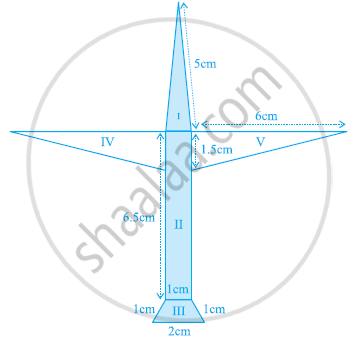Exercise 12.2 | Q 4 | Page 206

A triangle and a parallelogram have the same base and the same area. If the sides of triangle are 26 cm, 28 cm and 30 cm, and the parallelogram stands on the base 28 cm, find the height of the parallelogram.

Exercise 12.2 | Q 5 | Page 207

A rhombus shaped field has green grass for 18 cows to graze. If each side of the rhombus is 30 m and its longer diagonal is 48 m, how much area of grass field will each cow be getting?

Exercise 12.2 | Q 6 | Page 207

An umbrella is made by stitching 10 triangular pieces of cloth of two different colours (see the given figure), each piece measuring 20 cm, 50 cm and 50 cm. How much cloth of each colour is required for the umbrella?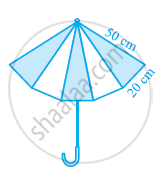Exercise 12.2 | Q 7 | Page 207

A kite in the shape of a square with a diagonal 32 cm and an isosceles triangles of base 8 cm and sides 6 cm each is to be made of three different shades as shown in the given figure. How much paper of each shade has been used in it?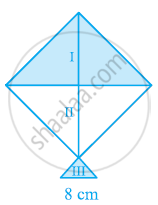Exercise 12.2 | Q 8 | Page 207

A floral design on a floor is made up of 16 tiles which are triangular, the sides of the triangle being 9 cm, 28 cm and 35 cm (see the given figure). Find the cost of polishing the tiles at the rate of 50p per cm2.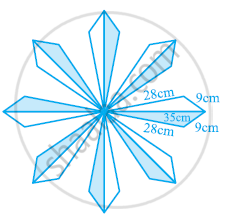Exercise 12.2 | Q 9 | Page 207

A field is in the shape of a trapezium whose parallel sides are 25 m and 10 m. The non-parallel sides are 14 m and 13 m. Find the area of the field.

## Chapter 12: Heron's Formula

Exercise 12.1Exercise 12.2## NCERT solutions for Class 9 Mathematics Textbook chapter 12 - Heron's Formula

NCERT solutions for Class 9 Mathematics Textbook chapter 12 (Heron's Formula) include all questions with solution and detail explanation. This will clear students doubts about any question and improve application skills while preparing for board exams. The detailed, step-by-step solutions will help you understand the concepts better and clear your confusions, if any. Shaalaa.com has the CBSE Class 9 Mathematics Textbook solutions in a manner that help students grasp basic concepts better and faster.

Further, we at Shaalaa.com provide such solutions so that students can prepare for written exams. NCERT textbook solutions can be a core help for self-study and acts as a perfect self-help guidance for students.

Concepts covered in Class 9 Mathematics Textbook chapter 12 Heron's Formula are Area of a Triangle by Heron's Formula, Application of Heron’s Formula in Finding Areas of Quadrilaterals, Area of a Triangle.

Using NCERT Class 9 solutions Heron's Formula exercise by students are an easy way to prepare for the exams, as they involve solutions arranged chapter-wise also page wise. The questions involved in NCERT Solutions are important questions that can be asked in the final exam. Maximum students of CBSE Class 9 prefer NCERT Textbook Solutions to score more in exam.

Get the free view of chapter 12 Heron's Formula Class 9 extra questions for Class 9 Mathematics Textbook and can use Shaalaa.com to keep it handy for your exam preparation

Advertisement Remove all ads
Share
Notifications

View all notifications

Forgot password?